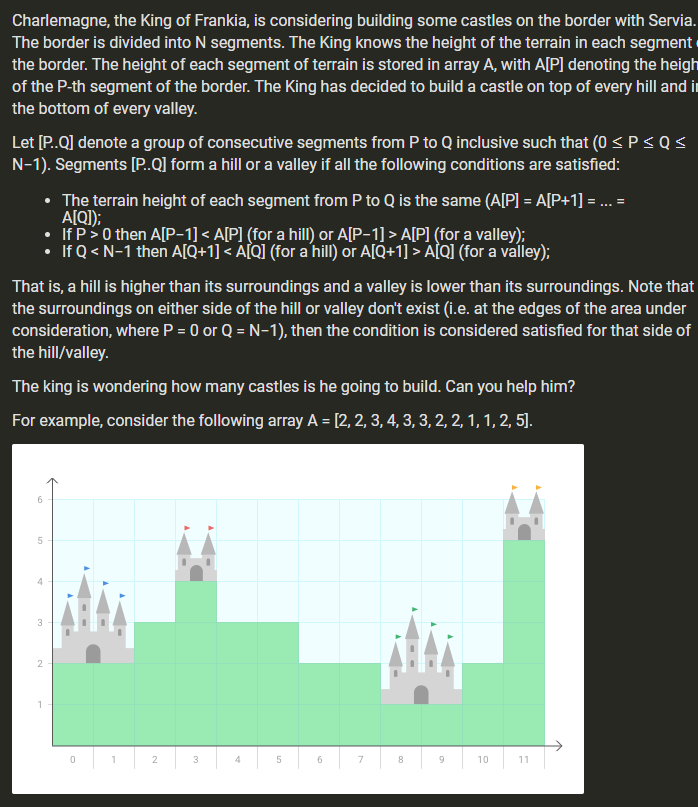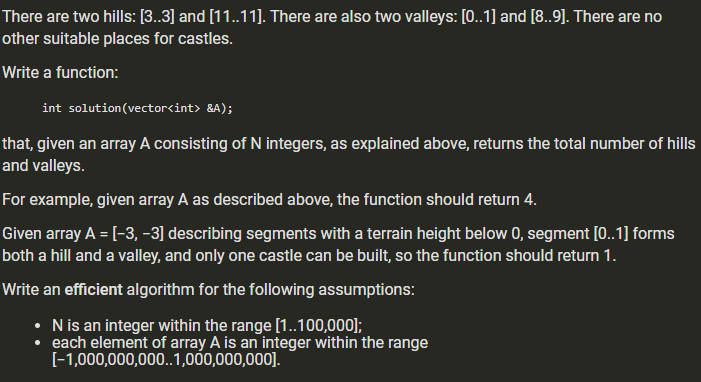# (Solved) : Charlemagne King Frankia Considering Building Castles Border Servia Border Divided N Segme Q42948824 . . .

\$9.00Charlemagne, the King of Frankia, is considering building some castles on the border with Servia. The border is divided into N segments. The king knows the height of the terrain in each segment the border. The height of each segment of terrain is stored in array A, with A[P] denoting the heigh of the P-th segment of the border. The King has decided to build a castle on top of every hill and in the bottom of every valley. Let [P..Q] denote a group of consecutive segments from P to Q inclusive such that (0 <PSIS N-1). Segments (P..Q] form a hill or a valley if all the following conditions are satisfied: • The terrain height of each segment from P to Q is the same (A[P] = A[P+1] = … = A[Q]); • If P>0 then A[P-1] <A[P] (for a hill) or A[P-1] > A[P] (for a valley); • If Q<N-1 then A[Q+1] <A[Q] (for a hill) or A[Q+1] > AQ (for a valley); That is, a hill is higher than its surroundings and a valley is lower than its surroundings. Note that the surroundings on either side of the hill or valley don’t exist (i.e. at the edges of the area under consideration, where P = 0 or Q = N-1), then the condition is considered satisfied for that side of the hill/valley. The king is wondering how many castles is he going to build. Can you help him? For example, consider the following array A = [2, 2, 3, 4,3,3, 2, 2, 1, 1, 2, 5). 0 1 2 3 4 5 6 7 8 9 10 11 There are two hills: [3..3] and [11..11). There are also two valleys: [0..1] and [8.9). There are no other suitable places for castles. Write a function: int solution (vector<int> &A); that, given an array A consisting of N integers, as explained above, returns the total number of hills and valleys. For example, given array A as described above, the function should return 4. Given array A =(-3, -3) describing segments with a terrain height below 0, segment [0..1) forms both a hill and a valley, and only one castle can be built, so the function should return 1. Write an efficient algorithm for the following assumptions: • N is an integer within the range (1..100,000); • each element of array A is an integer within the range (-1,000,000,000..1,000,000,000). Show transcribed image text Charlemagne, the King of Frankia, is considering building some castles on the border with Servia. The border is divided into N segments. The king knows the height of the terrain in each segment the border. The height of each segment of terrain is stored in array A, with A[P] denoting the heigh of the P-th segment of the border. The King has decided to build a castle on top of every hill and in the bottom of every valley. Let [P..Q] denote a group of consecutive segments from P to Q inclusive such that (0 0 then A[P-1] A[P] (for a valley); • If Q